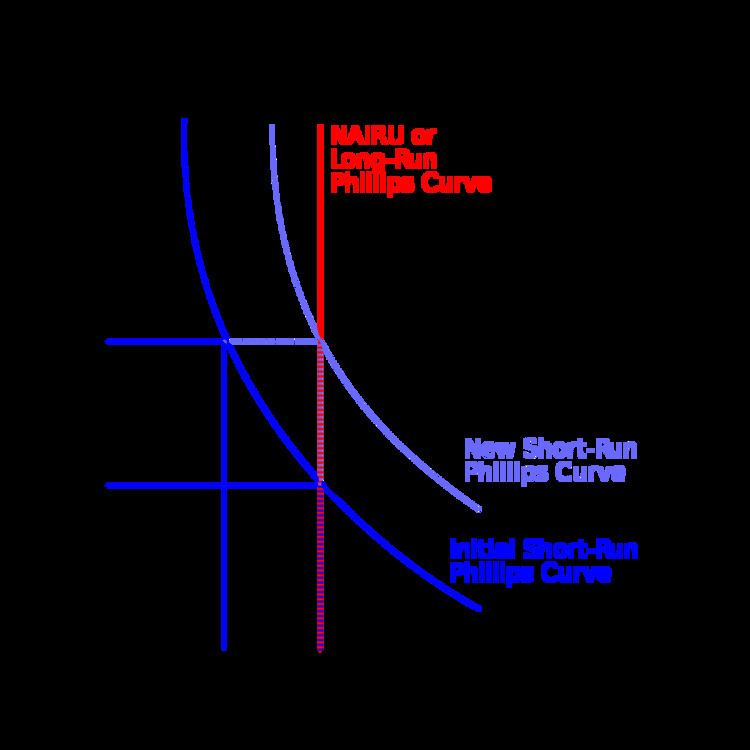# Elasticity (economics)

Updated on
Covid-19In economics, elasticity is the measurement of how responsive an economic variable is to a change in another. It gives answers to questions such as:

## Contents

• "If I lower the price of a product, how much more will sell?"
• "If I raise the price of one good, how will that affect sales of this other good?"
• "If the market price of a product goes down, how much will that affect the amount that firms will be willing to supply to the market?"
• An elastic variable (with elasticity value greater than 1) is one which responds more than proportionally to changes in other variables. In contrast, an inelastic variable (with elasticity value less than 1) is one which changes less than proportionally in response to changes in other variables. A variable can have different values of its elasticity at different starting points: for example, the quantity of a good supplied by producers might be elastic at low prices but inelastic at higher prices, so that a rise from an initially low price might bring on a more-than-proportionate increase in quantity supplied while a rise from an initially high price might bring on a less-than-proportionate rise in quantity supplied.

Elasticity can be quantified as the ratio of the percentage change in one variable to the percentage change in another variable, when the latter variable has a causal influence on the former. A more precise definition is given in terms of differential calculus. It is a tool for measuring the responsiveness of one variable to changes in another, causative variable. Elasticity has the advantage of being a unitless ratio, independent of the type of quantities being varied. Frequently used elasticities include price elasticity of demand, price elasticity of supply, income elasticity of demand, elasticity of substitution between factors of production and elasticity of intertemporal substitution.

Elasticity is one of the most important concepts in neoclassical economic theory. It is useful in understanding the incidence of indirect taxation, marginal concepts as they relate to the theory of the firm, and distribution of wealth and different types of goods as they relate to the theory of consumer choice. Elasticity is also crucially important in any discussion of welfare distribution, in particular consumer surplus, producer surplus, or government surplus.

In empirical work an elasticity is the estimated coefficient in a linear regression equation where both the dependent variable and the independent variable are in natural logs. Elasticity is a popular tool among empiricists because it is independent of units and thus simplifies data analysis.

A major study of the price elasticity of supply and the price elasticity of demand for US products was undertaken by Hendrik S. Houthakker and Lester D. Taylor.

## Elasticities of supply

Price elasticity of supply

The price elasticity of supply measures how the amount of a good that a supplier wishes to supply changes in response to a change in price. In a manner analogous to the price elasticity of demand, it captures the extent of horizontal movement along the supply curve relative to the extent of vertical movement. If the price elasticity of supply is zero the supply of a good supplied is "totally inelastic" and the quantity supplied is fixed.

Elasticities of scale

Elasticity of scale or output elasticity measures the percentage change in output induced by a collective percent change in the usages of all inputs. A production function or process is said to exhibit constant returns to scale if a percentage change in inputs results in an equal percentage in outputs (an elasticity equal to 1). It exhibits increasing returns to scale if a percentage change in inputs results in greater percentage change in output (an elasticity greater than 1). The definition of decreasing returns to scale is analogous.

## Elasticities of demand

Price elasticity of demand

Price elasticity of demand is a measure used to show the responsiveness, or elasticity, of the quantity demanded of a good or service to a change in its price. More precisely, it gives the percentage change in quantity demanded in response to a one percent change in price (ceteris paribus, i.e. holding constant all the other determinants of demand, such as income).

Cross-price elasticity of demand

Cross-price elasticity of demand is a measure of the responsiveness of the demand for one product to changes in the price of a different product. It is the ratio of percentage change in the former to the percentage change in the latter. If it is positive, the goods are called substitutes because a rise in the price of the other good causes consumers to substitute away from buying as much of the other good as before and into buying more of this good. If it is negative, the goods are called complements.

## Applications

The concept of elasticity has an extraordinarily wide range of applications in economics. In particular, an understanding of elasticity is fundamental in understanding the response of supply and demand in a market.

Some common uses of elasticity include:

• Effect of changing price on firm revenue. See Markup rule.
• Analysis of incidence of the tax burden and other government policies. See Tax incidence.
• Income elasticity of demand, used as an indicator of industry health, future consumption patterns and as a guide to firms' investment decisions. See Income elasticity of demand.
• Effect of international trade and terms of trade effects. See Marshall–Lerner condition and Singer–Prebisch thesis.
• Analysis of consumption and saving behavior. See Permanent income hypothesis.
• Analysis of advertising on consumer demand for particular goods. See Advertising elasticity of demand
• ## Variants

In some cases the discrete (non-infinitesimal) arc elasticity is used instead. In other cases, such as modified duration in bond trading, a percentage change in output is divided by a unit (not percentage) change in input, yielding a semi-elasticity instead.

## References

Similar Topics
Rob Baxter
Francis Moze
Bill Simmons
Topics